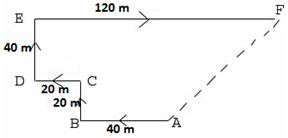# RRB NTPC 2021 Exam Memory Based Reasoning Questions with Answers (Phase-2): Check General Intelligence & Reasoning Questions asked in RRB NTPC 2021 CBT

RRB NTPC 2021 Exam Memory Based Reasoning Questions with Answers (Phase-2): Get the memory based questions from the Reasoning Section that came in RRB NTPC 2020 Exam held in online mode from 16th Jan to 30th Jan 2021.

Created On: Jan 28, 2021 11:10 ISTRRB NTPC 2021 Exam Memory Based Reasoning Questions with Answers (Phase-2): Check General Intelligence & Reasoning Questions asked in RRB NTPC 2021 CBT

RRB NTPC 2021 Exam Memory Based Reasoning Questions with Answers (Phase-2): RRB NTPC 2021 Phase-2 CBT Exam has been commenced from 16th January 2021 and will be conducted till 30th January 2021 for around 27 lakh Candidates across different exam centres in India. RRB NTPC 2021 Phase-3 Exam will be conducted from 31st Jan to 12th Feb 2021 for around 28 Lakh candidates. Computer Based Test (CBT) is being conducted for the recruitment of RRB Non-Technical Popular Categories (NTPC) 35281 Vacancies (Graduate & Under-Graduate Posts) in two shifts.

In this article, we are going to share the important memory based General Intelligence & Reasoning section Questions as per the feedback received by the candidates who have appeared for RRB NTPC 2021 Phase-2 CBT Exam. Candidates are advised to definitely cover these questions for scoring high marks in the Exam. Let’s have a look at the Important Questions that are being covered in the RRB NTPC 2021 Exam:

 RRB NTPC 2021 Reasoning Section Memory Based Questions with Answer

1. 12: 20 :: 30: __?

1. 40
2. 42
3. 50
4. 44

Answer: b)

Explanation:   12 is 32+ 3

20 is 42+4

30 is 52+5

and 62+6=42

2. Kidney: Nephrology :: Brain: __? __

1. Cardiology
2. Physiology
3. Anatomy
4. Neurology

Answer: d)

Explanation: Study of Kidney is Nephrology and the study of brain is called Neurology.

3. In a certain code ‘415’ means ‘milk is hot’; ‘18’ means ‘hot soup’; and ‘895’ means ‘soup is brown’. What number will indicate the word ‘brown’?

1. 9
2. 8
3. 5
4. 4

Answer: a)

Explanation: The code for ‘hot’ is 1. So, the code of ‘soup’ is 8. Now, the code for ‘is’ is 5. Hence, we can say that the code for ‘brown’ is 9.

4. If CEJQ is coded as XVQJ, then BDIP will be coded as:

1. WURQ
2. YWRK
3. WUPI
4. YWPI

Answer: b)

Explanation:   The first 13 letters of the alphabet are coded by the 13 letters of the alphabet in reverse, i.e.

= A B C D E F G H I J K L M (first 13 letters)

= Z Y X W V U T S R Q P O N (13 letters in reverse)

It is obvious from the above coding scheme that:

B = Y, D = W, I = R and K = P or P = K

Therefore, B D I P will be coded as Y W R K.

5. Ram facing south. He turns right and walks 40 metre. Then he turns right again and walks 20 metre. Then he turns left and walks 20 metre and then turning right walk 40 metre. Then he turns right again and walks 120 metre. In which direction he is from the starting point?

1. North-East
2. North-West
3. North
4. West

Answer: a)

Explanation:6. Read the following information carefully and answer the questions given below it:

E x K, means E is brother of K.

E – K, means E is mother of K.

E % K, means E is father of k.

Which of the following represents that’ N’ is the son of ’P ‘?

1. N x R %P
2. N%R x P
3. P % N x R
4. N-R % T

Answer: c)

Explanation: P%N, means P is father of N and N x R, means N is brother of R, thus N is the son of P.

7. 37, 10, 79, 16, 48, ?

1. 22
2. 20
3. 12
4. 18

Answer: c)

Explanation: Every second number is the sum of the digits of its previous number.

8. Choose the odd one:

1. 235
2. 342
3. 426
4. 819

Answer: b)

Explanation: Except the number 342, in all the numbers the sum of the first two digits is equal to the third digit.

9. Choose the odd one:

1. IEG
2. FBD
3. CWY
4. NJL

Answer: c)

Explanation: In all other groups, the second, the third and the first letters are alternate letters in the English alphabet.

10. The following question has a statement followed by two assumptions. Understand which of the conclusions are implicit in the statement and choose your answer options accordingly:

1. If only assumption I is implicit
2. If only assumption II is implicit
3. If neither I nor II is implicit
4. If both I and II are implicit

Statement: The use of internet has grown by 120% in over the last 5 years.

Assumptions:

1. There is an increase in sales of internet connection plans.
2. Televisions are not so informative medium for the senior citizens.

Answer: a)

Explanation: The statement doesn’t focus on televisions or senior citizens. Conclusion I can be implicit in the statement.

Comment ()

1 + 3 =
Post